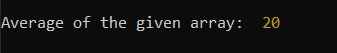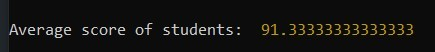# Collect.js | avg() Method

• Last Updated : 15 May, 2020

Collect.js is a fluent and convenient wrapper for working with arrays and objects. The JavaScript array is first transformed into a collection and then the function is applied to the collection.

The avg() method returns the average of all the items in a collection.
Installation:

• Collect.js can be installed via NPM:
`npm install --save collect.js`
• You can also use CDN of collect.js
`<script src="https://cdnjs.com/libraries/collect.js"></script>`

Syntax:

`collect(array).avg()`

Parameters: The collect() takes one argument that is converted into the collection and then avg() function is applied on it, which can take element if you apply it on the collection of objects.

Return Value: Returns a number which is average of the collection.

Below example illustrate the avg() method in JavaScript:

Example 1: Here collect = require(‘collect.js’) is used to import the collect.js library into the file.

 `const collect = require(``'collect.js'``); ` ` `  `let arr = [10, 20, 30]; ` ` `  `let average = collect(arr).avg(); ` ` `  `console.log(``"Average of the given array: "``, average); `

OutputExample 2: Array of objects.

 `const collect = require(``'collect.js'``); ` ` `  `let arr = [ ` `    ``{ ` `      ``name: ``'Rahul'``, ` `      ``score: 98, ` `    ``}, ` `    ``{ ` `      ``name: ``'Aditya'``, ` `      ``score: 96, ` `    ``}, ` `    ``{ ` `        ``name: ``'Abhishek'``, ` `        ``score: 80 ` `    ``}, ` `  ``]; ` ` `  `// converting object to collection ` `const collection = collect(arr); ` `   `  `// finding the average of all the score ` `let averageScore = collection.avg(``'score'``); ` `   `  `console.log(``"Average score of students: "``, averageScore); `

Output:Reference: https://collect.js.org/api/avg.html

My Personal Notes arrow_drop_up
Recommended Articles
Page :